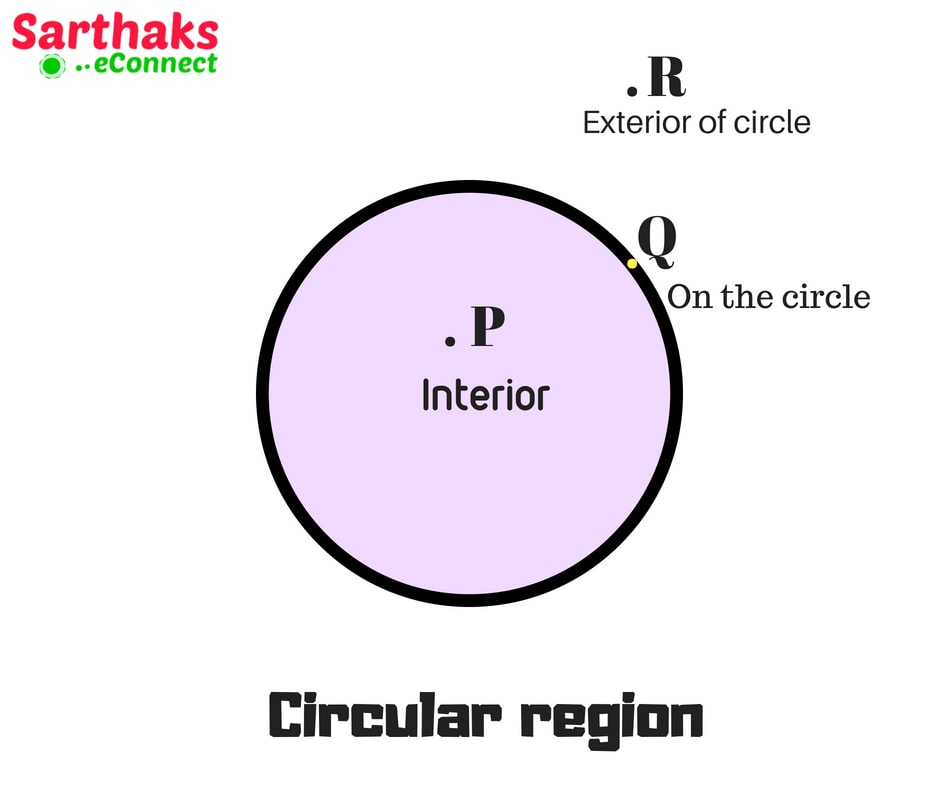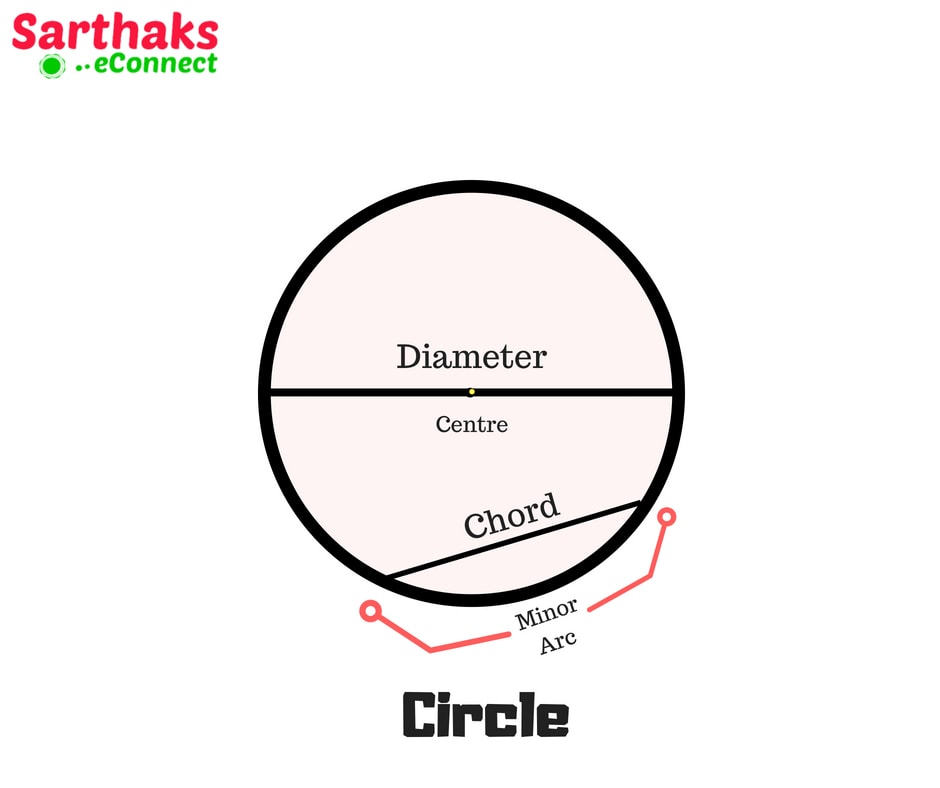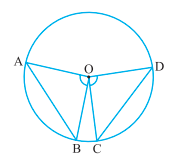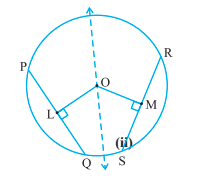#Also read, Circle Formulas Lines and Angles Coordinate Geometry Straight Line Conic Section Formula

# Circle Theorems

When you started reading circle for the first time you thought that this chapter is useless. It has no importance.

But the reality is something different. Think about the “wheel”. If there were no wheel then where the world which you are seeing now would be. Then you will get to know about the importance of circle. Circle consists of an important part of mathematics in geometry section. Circle theorems are stated in class 9.

When you look around, you will see many things which consist of circular interface. Some examples are coins, bottles, utensils, dining table etc.

## Circle:

Circle is the collection of all the points in a plane, which are at a fixed distance from a fixed point in that plane. In this section, we will study circle theorems.

• The fixed point is called the centre of the circle.
• The fixed distance is called the radius of the circle.A circle divides the constituent plane into 3 parts:1. Interior of circle
2. On the circle
3. Exterior of circle

See the given circle: Point ‘P’ is in the interior of circle, ‘Q’ is on the circle, ‘R’ is at the exterior of circle.

Before reading circle theorems understand some terminology.

## The terminology of circle:• Chord: It is the line joining any two points of the circle.
• Diameter: Chord passing through centre is diameter. It is the largest chord of circle. All diameters are of equal length. It is twice the radius.
• Arc: An arc is a piece of a circle between two points.
• Semicircle: Diameter divides the circle into two equal arcs which are semicircles.
• Circumference: The length of the complete circle is its circumference.
• Segment: The region between a chord and arcs is segment of circle.Sector: The region between an arc and the two radii, joining the centre to the endpoints of the arc is sector.

## Circle theorems and properties:

1. Equal chords of a circle subtends Equal angle at the centre.∠AOB = ∠COD
2. If the angles subtended by the chords of a circle at the centre are equal, then the chords are equal.
3. The perpendicular from the centre of a circle to a chord bisects the chord.AM = BM
4. The line drawn through the centre of a circle to bisect a chord is perpendicular to the chord.
5. There is one and only one circle passing through three given non-collinear points (not all lie on a single plane). The circle passing through these 3 points is called Radius involved is circumradius and centre of circle is circumcentre.6. Equal chords of a circle are equidistant from the centre. Also, equal chords of congruent circles are equidistant from their centres.7. Chords equidistant from the centre of a circle are equal in length.
8. If two chords of a circle are equal, then their corresponding arcs are congruent and conversely, if two arcs are congruent, then their corresponding chords are equal.+
9. Congruent arcs (or equal arcs) of a circle subtend equal angles at the centre.+
10. The angle subtended by an arc at the centre is double the angle subtended by it at any point on the remaining part of the circle.11. Angles in the same segment of a circle are equal.
12. If a line segment joining two points subtends equal angles at two other points lying on the same side of the line containing the line segment, the four points lie on a circle (i.e. they are concyclic).
13. Angle in a semicircle is a right angle.+